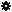﻿ Object Model: NurbsSurfaceMesh.GetSubSurfaceIndexArray

NurbsSurfaceMesh.GetSubSurfaceIndexArray

## NurbsSurfaceMesh.GetSubSurfaceIndexArrayv5.0

### Description

Returns the subsurface indices on which point locators are defined.

Notice that this information is part of the NurbsSurfaceMesh point locator's definition, and depends on the topology only (won't change if the geometry is deformed).

The normalized UV parameters within the subsurface can be queried with NurbsSurfaceMesh.GetNormalizedUVArray.

### C# Syntax

 ```Object NurbsSurfaceMesh.GetSubSurfaceIndexArray( PointLocatorData in_pPtLocators, Object in_pPointLocatorsIndices ); ```

### Scripting Syntax

 ```oArray = NurbsSurfaceMesh.GetSubSurfaceIndexArray( PtLocators, [PointLocatorsIndices] ); ```

### Return Value

1-dimensional Array of point locator indices

### Parameters

Parameter Type Description
PtLocators PointLocatorData Point locators to be described.
PointLocatorsIndices 1-dimensional Array Indices of the point locators to be described (all if not specified).

### Examples

#### JScript Example

 ```/* This example topologically describes the point locators resulting from the shrink-wrapping of a cube onto a NURBS surface sphere. */ NewScene( null, false ); var root = Application.ActiveSceneRoot; var CubePositionArray = root.AddGeometry("Cube", "MeshSurface").ActivePrimitive.Geometry.Points.PositionArray; var NurbsSphereGeom = root.AddGeometry("Sphere", "NurbsSurface").ActivePrimitive.Geometry; var CubeOnSpherePointLocators = NurbsSphereGeom.GetClosestLocations(CubePositionArray); var PtLocIndices = new Array(1); for(i = 0; i < CubeOnSpherePointLocators.Count; i++) { PtLocIndices = i; var PtLocSubSurf = NurbsSphereGeom.GetSubSurfaceIndexArray( CubeOnSpherePointLocators, PtLocIndices).toArray(); var PtLocUVs = NurbsSphereGeom.GetNormalizedUVArray( CubeOnSpherePointLocators, PtLocIndices).toArray(); logmessage("Point locator " + i + " is on subsurface " + PtLocSubSurf + " at {U = " + PtLocUVs + " , V = " + PtLocUVs + "}."); } // Expected results: //INFO : Point locator 0 is on subsurface 0 at {U = 1.3552785236419922e-16 , V = 0.3042299747467041}. //INFO : Point locator 1 is on subsurface 0 at {U = 0.75 , V = 0.3040647506713867}. //INFO : Point locator 2 is on subsurface 0 at {U = 2.4521901608032514e-17 , V = 0.6954541206359863}. //INFO : Point locator 3 is on subsurface 0 at {U = 0.75 , V = 0.6959285140037537}. //INFO : Point locator 4 is on subsurface 0 at {U = 0.25 , V = 0.3040647506713867}. //INFO : Point locator 5 is on subsurface 0 at {U = 0.5 , V = 0.3040647506713867}. //INFO : Point locator 6 is on subsurface 0 at {U = 0.25 , V = 0.6959285140037537}. //INFO : Point locator 7 is on subsurface 0 at {U = 0.5 , V = 0.6959285140037537}. ```